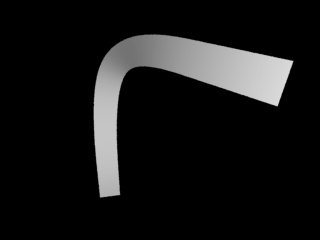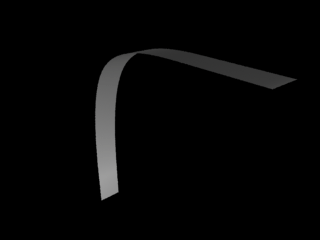### MtorRib Post Processingbefore post processing - figure 1after post processing - figure 2

##### Introduction

As of RenderMan Artist Tools version 11.5.3 the only attribute that can be assigned to a Maya curve using mtor is "constantwidth". Pixar's mtor plugin allows a curve to have a uniform width but not a sequence of width's or colors etc.

However, there are work-arounds. Both techniques involve post-processing the RIB files generated by Maya/mtor in such a way that the Curves statements are modified to include the "extra" attributes that we wish to assign a curve.

The difference between the work-arounds is that the simple one inserts extra curve data, say, widths or colors into a RIB file before it is rendered - see post processing curve widths. The disadvantage of this technique is that the data added to the curves in a RIB file cannot be directly controlled from "within" Maya.

This tutorial describes a more advanced (tricky would be a better) technique that relies on taking information from the Maya scene and "encoding" it into the names of the curves. In the example shown in this tutorial we will take information about the curve normals at points along each curve where V is 0.0, 0.33, 0.66 and 0.999. The RIB file(s) produced by Maya/mtor are post processed in such a way that the data previously "hidden" in the names of curves is de-coded and added to the curve.

##### Before and After

Before we implement this data-hiding technique lets look at a part of a RIB file before and after it has been post processed. The snippet of RIB shown below assumes we have run the MEL scripts shown in listings 1 and 2. The strange looking name, shown in bold, is the result of listing 1 encoding the data from normals at 4 locations sampled along the length of the curve.

 A Curves RIB statement from Maya/mtor ```AttributeBegin Attribute "identifier" "name" ["|curve1_p999_p001_n001..."] ConcatTransform [1 0 0 0 0 1 0 0 0 0 1 0 0 0 0 1] ShadingInterpolation "smooth" Basis "b-spline" 1 "b-spline" 1 Curves "cubic"  "nonperiodic" "P" [0 0 0 0 0 0 0 0 0 0 2 0 1.5 2 0 2 2 0 2 2 0 2 2 0] "constantwidth" [0.5] AttributeEnd ```

The normals are encoded to 3 decimal places. This seems to provide sufficient accuracy for most purposes while preventing the curve names from becoming too long.

 Curves statement after post processing ```AttributeBegin ConcatTransform [1 0 0 0 0 1 0 0 0 0 1 0 0 0 0 1] ShadingInterpolation "smooth" Basis "b-spline" 1 "b-spline" 1 Curves "cubic"  "nonperiodic" "P" [0 0 0 0 0 0 0 0 0 0 2 0 1.5 2 0 2 2 0 2 2 0 2 2 0] "constantwidth" [0.5] "N" [0.999 0.001 0.001 ...more xyz's] AttributeEnd ```

 listing 1 - addNormalsToCurve.mel ```//_________________________________________________ // encode //_________________________________________________ // Turn a float into a "coded" string. Note: only 3 // decimal places of accuracy. proc string encode(float \$f) { string \$out; if(\$f >= 0 && \$f < 0.001) \$out = "p001"; else if(\$f < 0 && \$f > -0.001) \$out = "n001"; else if(\$f > 0.999) \$out = "p999"; else if(\$f < -0.999) \$out = "n999"; else if(\$f < 0) \$out = "n" + abs(trunc(\$f * 1000)); else \$out = "p" + trunc(\$f * 1000); return \$out; } //_________________________________________________ // addNormalsToCurveName //_________________________________________________ // \$name must refer to a curve with 4 CV's. proc string addNormalsToCurveName(string \$name) { string \$newname = \$name; vector \$norm; for(\$i = 0; \$i < 4; \$i++) { \$norm[\$i] = `pointOnCurve -nn -pr (\$i * 0.333) \$name`; vector \$v = \$norm[\$i]; \$newname += "_" + encode(\$v.x); } rename -ignoreShape \$name \$newname; return \$newname; } ```

 listing 2 - makeCurves.mel ```//_________________________________________________ // length //_________________________________________________ // returns the length of a 2d vector proc float length(float \$a, float \$b) { return sqrt(\$a * \$a + \$b * \$b); } //_________________________________________________ // makeCurve //_________________________________________________ // Makes a single curve that "droops" down in Y by // an amount thats proportional to the length of the // curve in the X-Z plane. proc string makeCurve(vector \$v) { \$v = \$v + unit(sphrand(1) * 0.25); vector \$d = \$v/3; vector \$cv0 = <>, \$cv1 = \$d * 1; \$cv2 = \$d * 2; \$cv3 = \$d * 3; float \$len = length(\$v.x, \$v.z), \$droop3 = \$len * rand(2, 0.950), \$droop2 = \$len * 0.25, \$droop1 = \$len * 0.15, \$cv1 = <<\$cv1.x, \$cv1.y - \$droop1, \$cv1.z>>; \$cv2 = <<\$cv2.x, \$cv2.y - \$droop2, \$cv2.z>>; \$cv3 = <<\$cv3.x, \$cv3.y - \$droop3, \$cv3.z>>; string \$name = `curve -d 3 -p (\$cv0.x) (\$cv0.y) (\$cv0.z) -p (\$cv1.x) (\$cv1.y) (\$cv1.z) -p (\$cv2.x) (\$cv2.y) (\$cv2.z) -p (\$cv3.x) (\$cv3.y) (\$cv3.z)`; string \$shape[] = `listRelatives -s`; addAttr -ln mtorCurveWidth -dv 0.01 -k true \$shape; return addNormalsToCurveName(\$name); } //_________________________________________________ // makeCurves //_________________________________________________ // Makes a bunch of curves each of which "point" in // the general direction of vector \$v, with their bases // located around the (position) vector \$p. proc makeCurves(int \$num, vector \$v, vector \$p) { string \$items[]; for(\$n = 0; \$n < \$num; \$n++) \$items[\$n] = makeCurve(\$v); xform -t (\$p.x) (\$p.y) (\$p.z) \$items; }```
 Post processing a Maya/mtor RIB file consists of, reading the file line-by-line finding the Curves statements "de-coding" the curve name into 4 xyz's copying the xyz values, plus the other info, to a copy of the rib file Any general purpose scripting/programming language can be used to post-process RIB files. This tutorial provides an example TCL script (listing 2) that post process a single RIB file. Before continuing you may wish to review another tutorial dealing with file filtering.
 listing 2 - Tcl script ```proc decode { input } { if { [string equal [string index \$input 0] p] } { set out 0.[string trimleft \$input p] append out " " } else { set out -0.[string trimleft \$input n] append out " " } return \$out } proc processRib { inpath outpath } { set in [open \$inpath r] set out [open \$outpath w] while { [eof \$in] != 1 } { gets \$in line if { [llength \$line] == 4 && [string equal [lindex \$line 0] "Attribute"] && [string equal [lindex \$line 1] "identifier"] && [string equal [lindex \$line 2] "name"]} { set tokens [split [lindex \$line 3] _|] set N "\t\t\"N\" \[" # The coordinates are in 3rd to second to last token. for {set i 2} {\$i <= 11} {incr i 3} { set x [lindex \$tokens \$i] set y [lindex \$tokens [expr \$i + 1]] set z [lindex \$tokens [expr \$i + 2]] set xyz "[decode \$x][decode \$y][decode \$z]" # Because mtor repeats the first and last cv's # we must also repeat the first and last normals if {\$i == 2 || \$i == 11} { append N \$xyz\$xyz } else { append N \$xyz } } set N [string trimright \$N] append N "\]" # Now we have decoded the name we must look for AttributeEnd # so that we can copy the data to the output RIB file while { [eof \$in] != 1 } { gets \$in line if { [string equal [lindex \$line 0] "AttributeEnd"] } { puts \$out \$N puts \$out \$line break } else { puts \$out \$line } } } else { puts \$out \$line } } close \$in close \$out } set src PATH_TO_DIRECTORY/single_curve.rib set dst PATH_TO_DIRECTORY/single_curve_copy.rib processRib \$src \$dst```